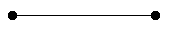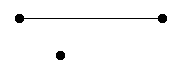SEARCH HOMEMath Central Quandaries & QueriesQuestion from Maftuna: A graph has 100 vertices and only one edge. How many connected components does it have?Hi,

A graph is a collection of vertices and edges where each edge connects two different vertices.

Let's start with a smaller example, say the graph has two vertices and one edge. The graph looks likeThe two vertices and edge are connected so this graph has one connected component.

Now suppose the graph has three vertices and one edge. The graph looks likeThe graph is now not connected, it is composed of two connected pieces and hence it has two connected components.

Now suppose the graph has four vertices and one edge. What does the graph looks like? How many connected components are there? What about 100 vertices and one edge?

PennyMath Central is supported by the University of Regina and The Pacific Institute for the Mathematical Sciences.